# NCERT Solutions for Class 10 Science Chemical Reactions and Equations Part 2## myCBSEguide App

CBSE, NCERT, JEE Main, NEET-UG, NDA, Exam Papers, Question Bank, NCERT Solutions, Exemplars, Revision Notes, Free Videos, MCQ Tests & more.

NCERT solutions for Class 10 Science Chemical Reactions and Equations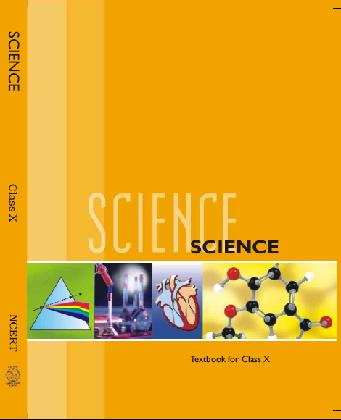## NCERT Class 10 Science Chapter wise Solutions

1. Chemical Reactions and Equations
2. Acids, Bases and Salts
3. Metals and Non-metals
4. Carbon and Its Compounds
5. Periodic Classification of Elements
6. Life Processes
7. Control and Coordination
8. How do Organisms Reproduce?
9. Heredity and Evolution
10. Light Reflection and Refraction
11. Human Eye and Colourful World
12. Electricity
13. Magnetic Effects of Electric Current
14. Sources of Energy
15. Our Environment
16. Management of Natural Resources

## NCERT Solutions for Class 10 Science Chemical Reactions and Equations Part 2

1. Which of the following statement about the reaction below are incorrect?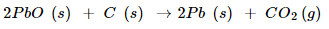(b) Carbon dioxide is getting oxidized

(c) Lead oxide is getting oxidized

i. (a) and (b)

ii. (a) and (c)

iii. (a), (b) and (c)

iv. All

Ans. As statement (a) and (b) are incorrect, answer (i) is correct.

NCERT Solutions for Class 10 Science Chemical Reactions and Equations Part 2

###### 2.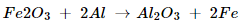The above reaction is an example of a

(a) combination reaction

(b) double displacement reaction

(c) decomposition reaction

(d) displacement reaction

Ans. This is an example of displacement reaction because Fe in FeO3 has been displaced by Al. Hence correct answer is (d).

### 3. What happens when dilute hydrochloric acid is added to iron filling? Tick the correct answer

(a) Hydrogen gas and iron chloride are produced.

(b) Chlorine gas and iron hydroxide are produced

(c) No reaction takes place

(d) Iron salt and water are produced

### 4. What is balanced chemical equation? Why should chemical equation be balanced?

Ans. The reaction in which the number of atoms of each element is equal on the reactant side and product side is called balanced equation.

Chemical reaction should be balanced because only a balanced equation tells us the relative quantities of different reactants and products involved in the reaction.

NCERT Solutions for Class 10 Science Chemical Reactions and Equations Part 2

### 5. Translate the following statements into chemical equations and then balance them.

(a) Hydrogen gas combines with nitrogen to form ammonia.

(b) Hydrogen sulphide gas burns in air to give water and Sulpher dioxide.

(c) Barium chloride reacts with aluminum sulphate to give aluminum chloride and precipitate of barium sulphate

Ans. (a).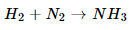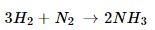(b).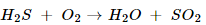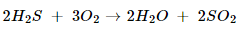(c).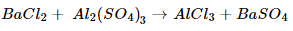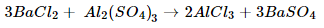(d).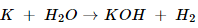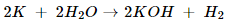NCERT Solutions for Class 10 Science Chemical Reactions and Equations Part 2

### 6. Balance the following chemical equations: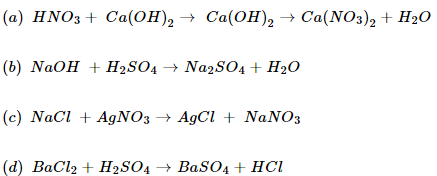Ans. Balanced chemical equation are: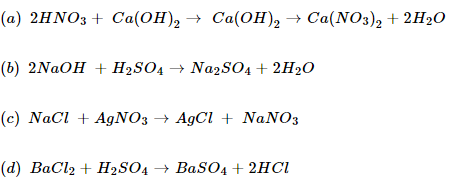NCERT Solutions for Class 10 Science Chemical Reactions and Equations Part 2

### 7. Write the balanced chemical equations for the following reactions.

(a) Calcium hydroxide + Carbon dioxide → Calcium carbonate + Water

(b) Zinc + Silver nitrate → Zinc nitrate + Silver

(c) Aluminum + Copper chloride → Aluminum chloride +Copper

(d) Barium chloride + Potassium sulphate → Barium sulphate + potassium chloride

Ans. Balanced chemical equation for reactions are: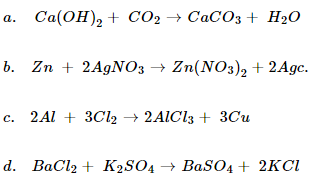NCERT Solutions for Class 10 Science Chemical Reactions and Equations Part 2

### 8. Write the balanced chemical equation for the following and identify the type of reaction in each case.

(a) Potassium bromide (s) + Barium iodide (aq) → Potassium iodide (aq) + Barium bromide(s)

(b) Zinc carbonate (s) → Zinc oxide (s) + Carbon dioxide (g)

(c) Hydrogen (g) + Chlorine (g) → Hydrogen chloride (g)

(d) Magnesium (s) + Hydrochloric acid (aq) → Magnesium chloride (aq) + Hydrogen (g)

Ans. Balanced equations are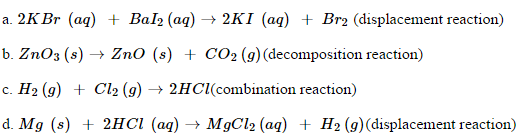### 9. What does one mean by exothermic and endothermic reactions? Give examples.

Ans. A reaction in which energy is released in the form of heat or light is called exothermic reaction. Example of exothermic reaction are: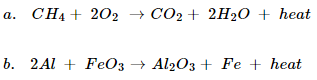A reaction in which energy is absorbed from the surrounding and cooling is produced is called endothermic reaction. Example of endothermic reaction are: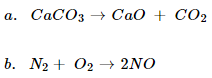NCERT Solutions for Class 10 Science Chemical Reactions and Equations Part 2

### 10. Why respiration is considered an exothermic reaction? Explain.

Ans. During respiration, we inhale oxygen from the atmosphere which reacts with glucose in your body cells to produce carbon dioxide and water.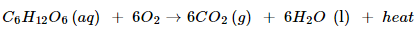Heat is liberated in this process; hence respiration is considered an exothermic reaction.

### 11. Why decomposition reactions are called the opposite of combination reactions? Write equations for these reactions.

Ans.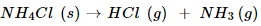In a decomposition reaction, a single substance breaks down into two or more substances while in a combination reaction, two or more substances react to produce one substance. Therefore, decomposition reactions are called opposite of combination reactions.

Example of decomposition reaction: Example of combination reaction: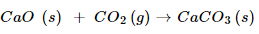NCERT Solutions for Class 10 Science Chemical Reactions and Equations Part 2

### 12. Write one equation each for decomposition reactions where energy is supplied in the form of heat, light or electricity.

Ans. Decomposition by heat: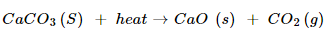Decomposition by electricity: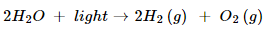Decomposition by light: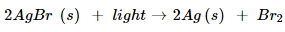### 13. What is difference between displacement and double displacement reactions? Write equations for these reactions.

Ans. In displacement reaction, more reactive element displaces the less reactive element from its compound. For example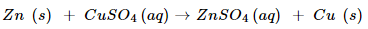But in double displacement reaction, exchange of ions takes place. For example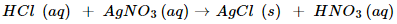NCERT Solutions for Class 10 Science Chemical Reactions and Equations Part 2

### 14. In refining of silver, the recovery of silver from silver nitrate solution involved displacement by copper metal. Write down the reaction involved.

Ans. The reaction involved is: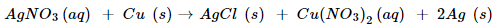### 15. What do you mean by a precipitation reaction? Explain by giving examples.

Ans. A chemical reaction in which an insoluble substance (precipitate) is formed is called precipitation reaction. For example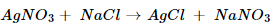NCERT Solutions for Class 10 Science Chemical Reactions and Equations Part 2

### 16. Explain the following in terms of gain and loss of oxygen with two examples each?

a. Oxidation

b. Reduction

Ans. Oxidation- addition of oxygen or removal of hydrogen in a chemical reaction is called oxidation reaction. For example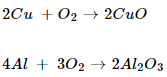Reduction- addition of hydrogen or removal of oxygen in a chemical reaction is called oxidation reaction. For example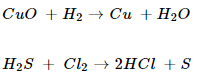NCERT Solutions for Class 10 Science Chemical Reactions and Equations Part 2

### 17. A shiny brown coloured element ‘X’ on heating in air becomes black in colour. Name the element ‘X’ and the black coloured compound formed.

Ans. The brown coloured element ‘X ‘ is copper. On heating in air it forms copper oxide, which is black in colour.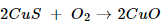### 18. Why do we apply paint on iron articles?

Ans. We apply paint on iron articles to prevent rusting. Iron articles do not come in contact of atmospheric oxygen and moisture and thus the rusting is prevented.

### 19. Oil and fat containing food items are flushed with nitrogen. Why?

Ans. Oil and fat containing items get rancid due to oxidation with atmospheric oxygen. To prevent rancidity food items are flushed with nitrogen. Nitrogen do not reacts with oil and fat containing items.

NCERT Solutions for Class 10 Science Chemical Reactions and Equations Part 2

### 20. Explain the following terms with one example each.

a. Corrosion

b. Rancidity.

Ans. Corrosion- action of air, water, acid or other substance on metal surface to form oxides and carbonates is called corrosion. Corrosion of iron is called rusting. Green coating on copper and black coating on silver is examples of corrosion.

Rancidity-change in smell of food item containing fat and oil when kept open for longer time due to oxidation is called rancidity. To prevent rancidity food items are flushed with nitrogen or kept in airtight containers.

## NCERT Solutions for Class 10 Science

NCERT Solutions Class 10 Science PDF (Download) Free from myCBSEguide app and myCBSEguide website. Ncert solution class 10 Science includes text book solutions from Book. NCERT Solutions for CBSE Class 10 Science have total 16 chapters. 10 Science NCERT Solutions in PDF for free Download on our website. Ncert Science class 10 solutions PDF and Science ncert class 10 PDF solutions with latest modifications and as per the latest CBSE syllabus are only available in myCBSEguide.

## CBSE app for Students

To download NCERT Solutions for class 10 Social Science, Computer Science, Home Science, Hindi , English, Maths Science do check myCBSEguide app or website. myCBSEguide provides sample papers with solution, test papers for chapter-wise practice, NCERT solutions, NCERT Exemplar solutions, quick revision notes for ready reference, CBSE guess papers and CBSE important question papers. Sample Paper all are made available through the best app for CBSE students and myCBSEguide website.